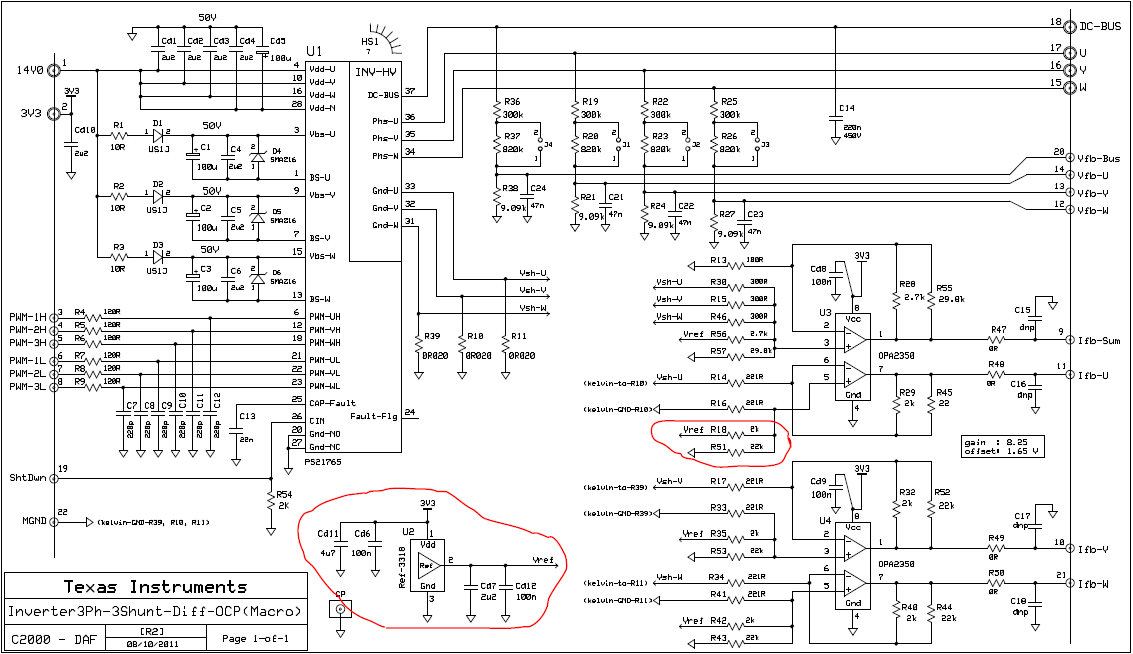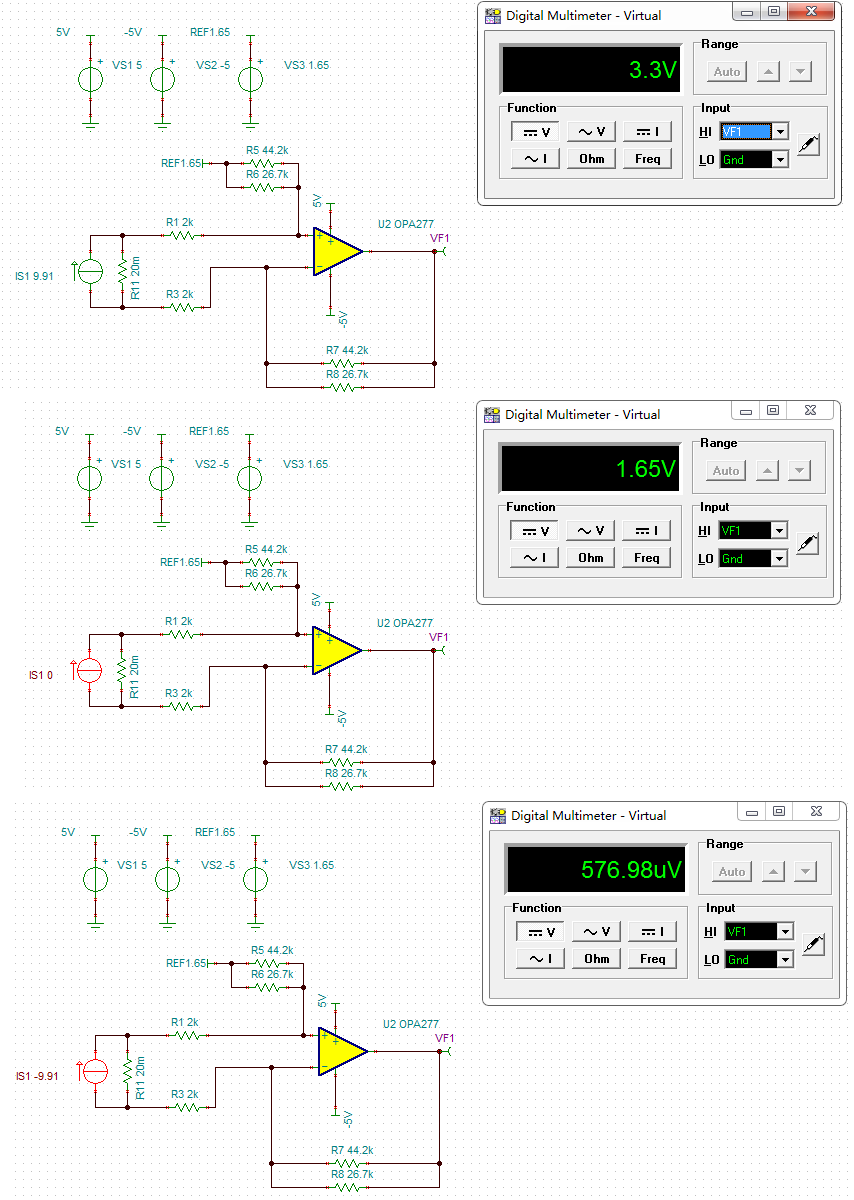If you have a related question, please click the "Ask a related question" button in the top right corner. The newly created question will be automatically linked to this question.

Part Number: TMDSHVMTRPFCKIT
Other Parts Discussed in Thread: REF3318

Dear team:

If the AD sampling range is from 0V to 3.3V, then the median value should be 1.65V. Why use 1.8V reference source to participate in the calculation in this circuit?As shown in U2 in the figure above, REF3318 is a 1.8V reference source. For comparison, I designed the following simulation circuit:As the pictrue shows above, Sampling resistance is also 0.02ohm, circuit amplification factor is 8.32 (R7 shunt R8, shunt resistance divided by 2K)
When the current flowing through the sampling resistance is 9.91 Amp, the operational amplifier output is 3.3V.
When the current flowing through the sampling resistor is 0.1mp, the output of the operational amplifier is 1.65V.
When the current flowing through the sampling resistance is negative 9.91 Amp, the output of the operational amplifier is 0V.
If we design the 1.8V reference REF3318 in HVMotorCtrl+PFC-SCH [R1.1], how can the following operational circuit realize offset of 1.65V?

Best regards